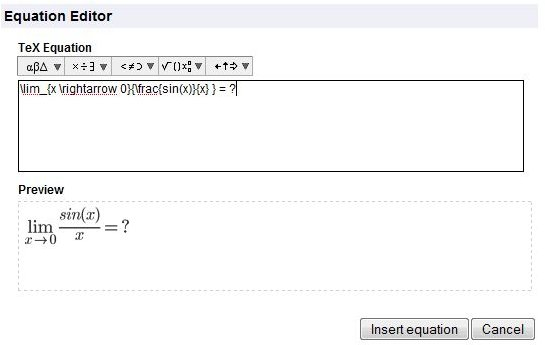# How to Use the Equation Editor in Docs

Page content

## How Do You Use the Google Docs Equation Editor?

The symbols and characters needed to express most mathematical equations are not available on a standard computer keyboard. The Google docs equation editor solves this problem by offering these characters. To use the equation editor, open a Google docs document. From within the document, choose the “insert” menu and select “equation.” This opens the equation editor. The equation editor’s drop down menu can be used to select various characters and symbols for creating the equation. Greek letters, a wide range of mathematical symbols and operands, arrows, brackets and script letters are all availavle choices from within the drop down menu. If you select a square root symbol, for example, Google docs equation editor inserts the square root formula into the document. The default variables in the square root equation can be changed within the editor. When finished editing the equation to specification, select “insert equation.”

## Editing or Deleting the Equation

The equation now appears in the document as an image. To edit or delete the image, simply highlight it with a mouse and click the highlighted image. A box will appear asking if the user wishes to edit or remove the image. Because it is inserted into Google docs as an image, it can also be enlarged or reduced by highlighting the image and dragging the corner of the highlighted box with a mouse. The document can be exported and imported into a word processing document, such as Microsoft Word. However, the equation cannot be edited outside of Google docs.

## The Equation Editor Also Accepts LaTeX CodeAlternatively, if a user is familiar with LaTeX code, this code can be typed directly into the Google docs equation editor. LaTeX is a document markup language used for creating formulas. LaTeX code is translated by the Google docs equation editor into images and symbols. This code can be very helpful when needing to add arrays or matrices to a document.

The Google docs equation editor can be useful to students and professionals who wish to collaborate on a document. Because the equation editor is new, advances in the functionality are sure to become available in the future as Google continues to tweak docs to make it more useful to its users.